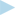Chapter 14

# Intro to Integration and Approximating Area

In This ChapterIntegrating — adding it all upApproximating areas and sizing up sigma sumsUsing the definite integral to get exact areasTotaling up trapezoidsSimpson’s rule: Calculus for Bart and Homer

Since you’re still reading this book, I presume that means you survived differentiation (Chapters 9 through 13). Now you begin the second major topic in calculus: integration. Just as two simple ideas lie at the heart of differentiation — rate (like miles per hour) and the steepness or slope of a curve — integration can also be understood in terms of two simple ideas — adding up small pieces of something and the area under a curve. In this chapter, I introduce you to these two fundamental concepts.